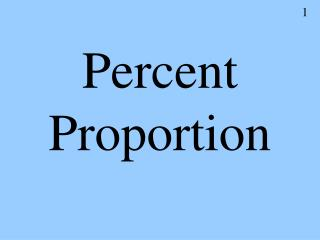DownloadDownload PresentationPercent Proportion

# Percent Proportion

Télécharger la présentation## Percent Proportion

- - - - - - - - - - - - - - - - - - - - - - - - - - - E N D - - - - - - - - - - - - - - - - - - - - - - - - - - -
##### Presentation Transcript

1. Percent Proportion

2. Percent • Means ‘of a hundred’

3. The Percent Proportion(s) • You will use a proportion to solve most percent problems. • Remember: the percent will always be related to a hundred.

4. = The Percent Proportion(s) ‘is’ is the number with the word ‘is’ ‘of’ is the number with the word ‘of’ is % The percent % is always over 100. 100 is always on the bottom. of 100 This proportion can be used when dealing with simple percent statements such as: “What is 10% of 100?” “10 is what percent of 100?” “10 is 10% of what number?”

5. = = is % of 100 50 is what percent of 230? • Use the proportion. 50 x 21.73913043 or x = 21.7% 50 is 21.7% of 230 230 100 Cross multiply to get the answer.

6. = = is % of 100 What is 85% of 40? Use the proportion. x 85 40 100 x = 34 34 is 85% of 40 Cross multiply to get the answer.

7. = = is % of 100 25% of what number is 400? Use the proportion. 400 25 x 100 x = 1600 25% of 1600 is 400 Cross multiply to get the answer.

8. = = is % of 100 What is 175% of 80? Use the proportion. x 175 80 100 Cross multiply to get the answer. x = 140 140 is 175% of 80

9. 19 is 25% of what number? • What percent of 256 is 64? • What is 45% of 178?

10. = = is % of 100 19 is 25% of what number? Use the proportion. 19 25 x 100 x = 76 19 is 25% of 76 Cross multiply to get the answer.

11. = = is % of 100 What percent of 256 is 64? Use the proportion. 64 x 256 100 x = 25% 25% of 256 is 64 Cross multiply to get the answer.

12. = = is % of 100 What is 45% of 178? Use the proportion. x 45 178 100 Cross multiply to get the answer. x = 80.1 80.1 is 45% of 178

13. Word Problems